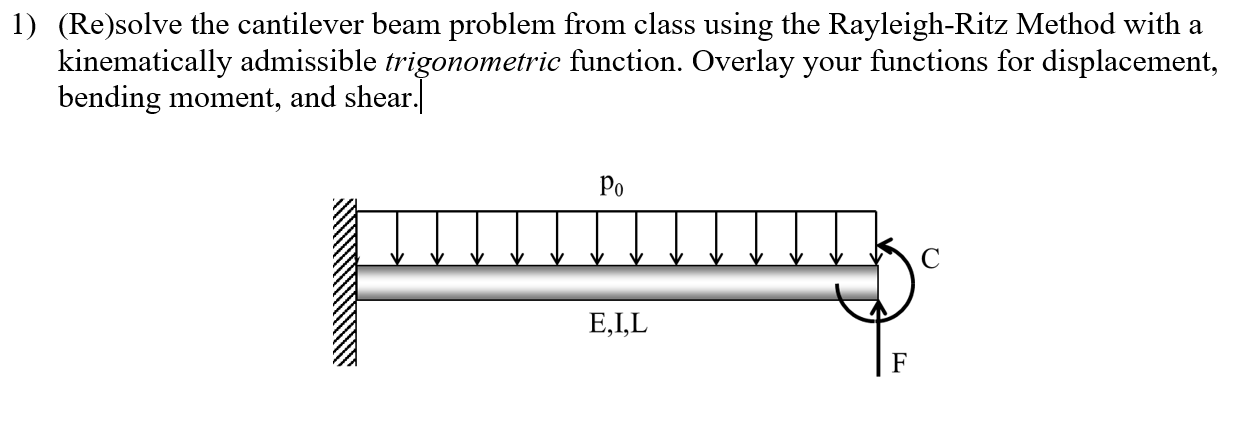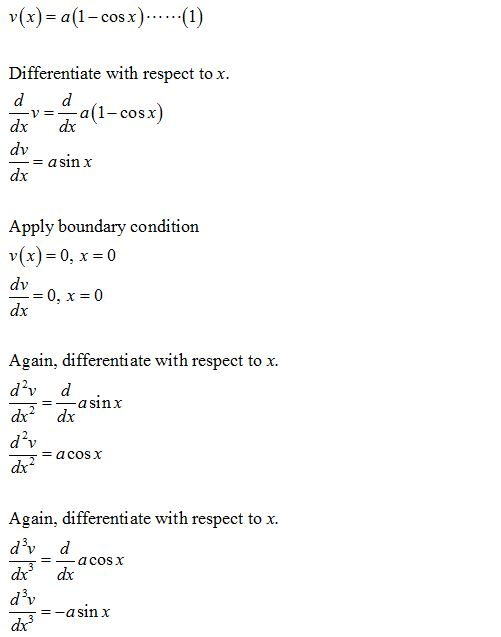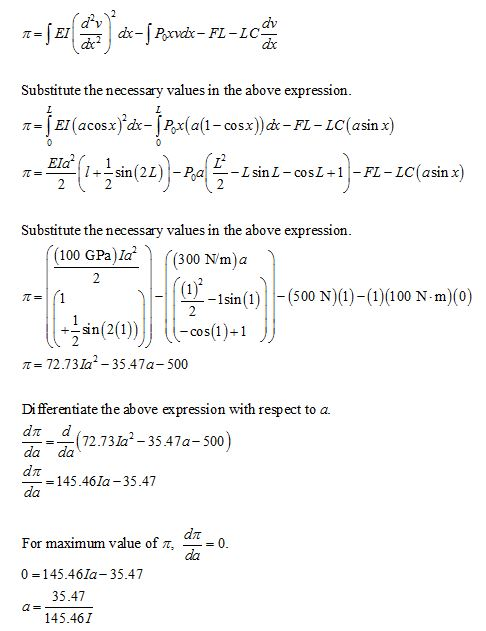# 1) (Re)solve the cantilever beam problem from class using the Rayleigh-Ritz Method with akinematically admissible trigonometric function. Overlay your functions for displacement,bending moment, and shear.PoE,I,L

Question
163 views

Given: L= 1m, F= 500N, c= 100N.m , E= 100Gpa, Po=300N/m

Assume v (×)= a( 1-cosx )help_outlineImage Transcriptionclose1) (Re)solve the cantilever beam problem from class using the Rayleigh-Ritz Method with a kinematically admissible trigonometric function. Overlay your functions for displacement, bending moment, and shear. Po E,I,L fullscreen
check_circle

Step 1

Write the expression of displacement function.Step 2

Write the expression of total potential energy....

### Want to see the full answer?

See Solution

#### Want to see this answer and more?

Solutions are written by subject experts who are available 24/7. Questions are typically answered within 1 hour.*

See Solution
*Response times may vary by subject and question.
Tagged in